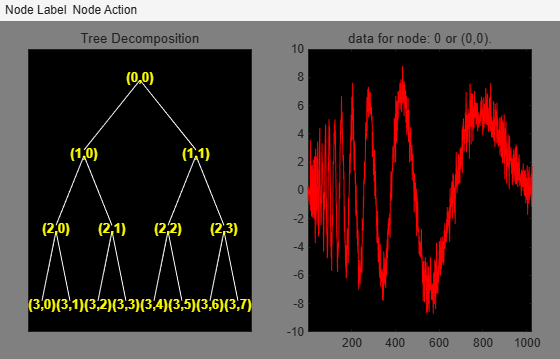wpdec

Wavelet packet decomposition 1-D

Description

tobj = wpdec(x,n,wname) returns a wavelet packet tree object tobj corresponding to the wavelet packet decomposition of the vector x at level n, using Shannon entropy and the wavelet specified by wname (see wfilters for more information).

example

tobj = wpdec(x,n,wname,etype,p) uses the entropy type specified by etype. p is an optional parameter depending on the value of etype. See wentropy for more information.

Note

tobj = wpdec(x,n,wname) is equivalent to tobj = wpdec(x,n,wname,'shannon').

Examples

collapse all

Decompose the signal at level 3 with db1 wavelet packets using Shannon entropy.

wpt = wpdec(noisdopp,3,'db1','shannon');

Plot the wavelet packet tree.

plot(wpt)Input Arguments

collapse all

Input data, specified as a real-valued numeric vector.

Data Types: single | double | int8 | int16 | int32 | int64 | uint8 | uint16 | uint32 | uint64

Decomposition level, specified as a positive integer.

Data Types: single | double

Wavelet used in the wavelet packet decomposition, specified as a character vector or string scalar. The wavelet is from one of the following wavelet families: Daubechies, Symlets, Fejér-Korovkin, Discrete Meyer, Biorthogonal, and Reverse Biorthogonal. See wfilters for the wavelets available in each family.

Entropy type, specified as one of the following:

Entropy Type (T)

Threshold Parameter (P)

'shannon'

P is not used.

'log energy'

P is not used.

'threshold'0 ≤ P

P is the threshold.

'sure'0 ≤ P

P is the threshold.

'norm'1 ≤ P

P is the power.

'user'Character vector

P is a character vector containing the file name of your own entropy function, with a single input x.

'FunName'No constraints on P

FunName is any character vector other than the previous entropy types listed.

FunName contains the file name of your own entropy function, with x as input and P as an additional parameter to your entropy function.

etype and the threshold parameter p together define the entropy criterion. See wentropy for more information.

Note

The 'user' option is historical and still kept for compatibility, but it is obsoleted by the last option described in the table above. The FunName option does the same as the 'user' option and in addition gives the possibility to pass a parameter to your own entropy function.

Threshold parameter, specified by a real number, character vector, or string scalar. p and the entropy type etype together define the entropy criterion.

collapse all

Wavelet Packet Decomposition

The wavelet packet method is a generalization of wavelet decomposition that offers a richer signal analysis. Wavelet packet atoms are waveforms indexed by three naturally interpreted parameters: position and scale as in wavelet decomposition, and frequency.

For a given orthogonal wavelet function, a library of wavelet packets bases is generated. Each of these bases offers a particular way of coding signals, preserving global energy and reconstructing exact features. The wavelet packets can then be used for numerous expansions of a given signal.

Simple and efficient algorithms exist for both wavelet packets decomposition and optimal decomposition selection. Adaptive filtering algorithms with direct applications in optimal signal coding and data compression can then be produced.

In the orthogonal wavelet decomposition procedure, the generic step splits the approximation coefficients into two parts. After splitting we obtain a vector of approximation coefficients and a vector of detail coefficients, both at a coarser scale. The information lost between two successive approximations is captured in the detail coefficients. The next step consists in splitting the new approximation coefficient vector; successive details are never re-analyzed.

In the corresponding wavelet packets situation, each detail coefficient vector is also decomposed into two parts using the same approach as in approximation vector splitting. This offers the richest analysis: the complete binary tree is produced in the one-dimensional case or a quaternary tree in the two-dimensional case.

Tips

• To obtain the wavelet packet transform of a 1-D multisignal, use dwpt.

Algorithms

The algorithm used for the wavelet packets decomposition follows the same line as the wavelet decomposition process (see dwt and wavedec for more information).

 Coifman, R.R., and M.V. Wickerhauser. “Entropy-Based Algorithms for Best Basis Selection.” IEEE Transactions on Information Theory 38, no. 2 (March 1992): 713–18. https://doi.org/10.1109/18.119732.

 Meyer, Yves. Les ondelettes. Algorithmes et applications, Colin Ed., Paris, 2nd edition, 1994. (English translation: Wavelets: Algorithms and Applications, SIAM).

 Wickerhauser, M.V. "INRIA lectures on wavelet packet algorithms." Proceedings ondelettes et paquets d'ondes, 17–21 June 1991, Rocquencourt, France, pp. 31–99.

 Wickerhauser, Mladen Victor. Adapted Wavelet Analysis from Theory to Software. Wellesley, MA: A.K. Peters, 1994.

Wavelet Toolbox Documentation